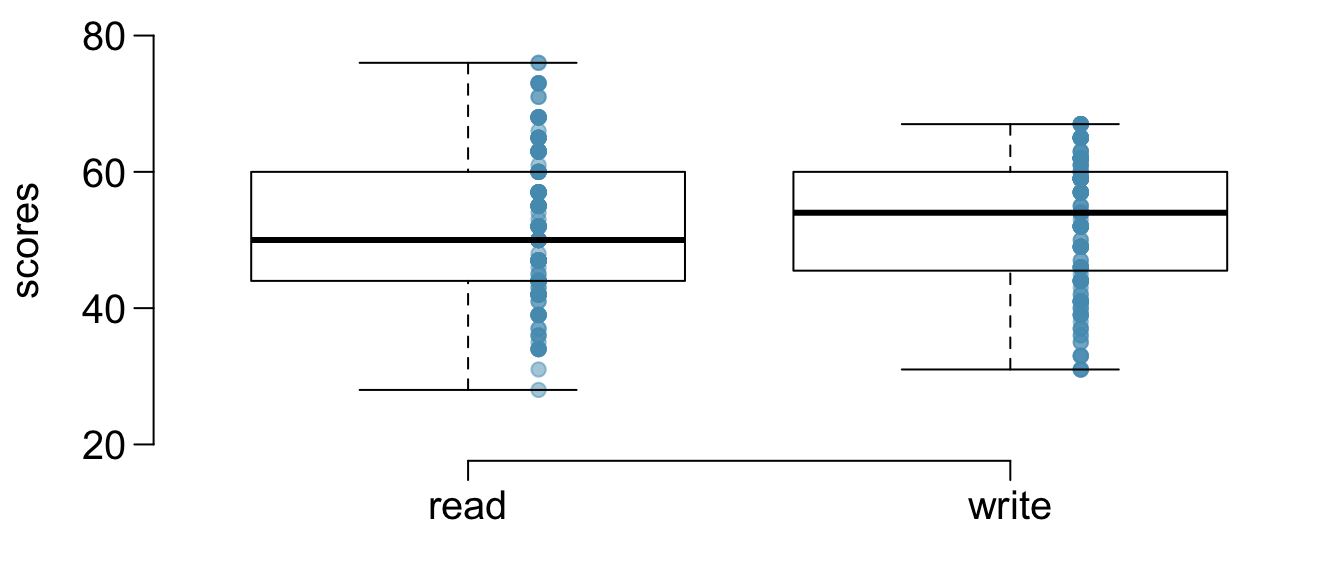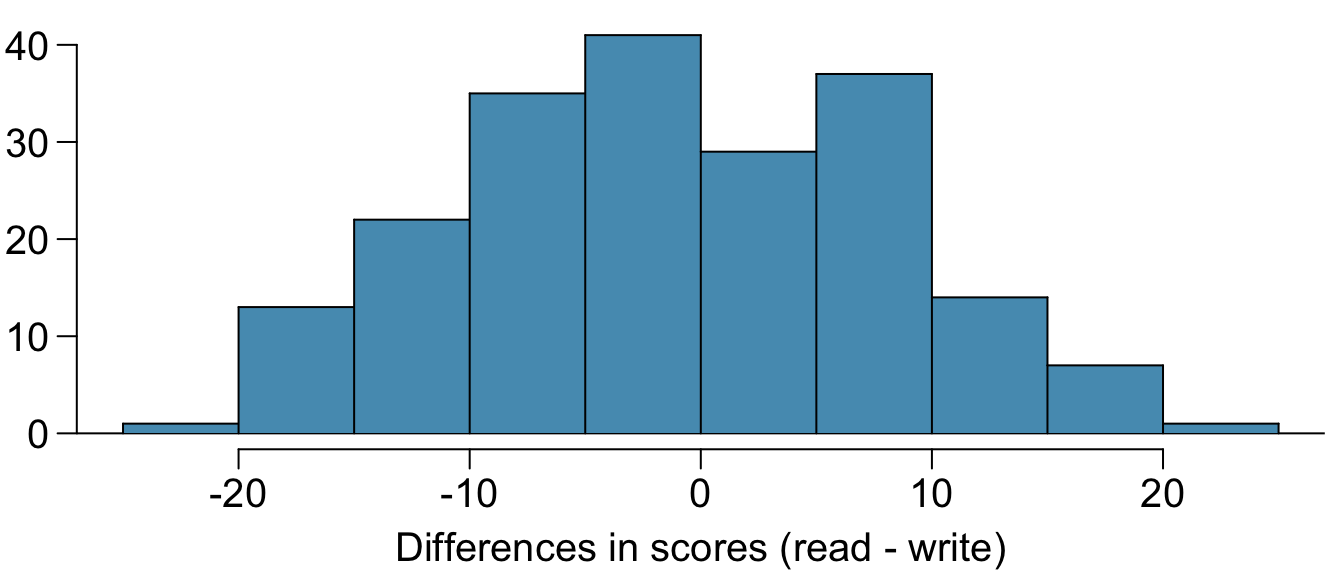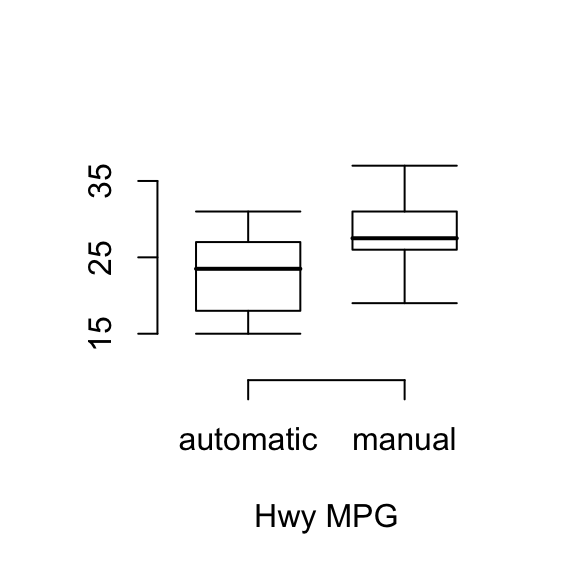knitr::opts_chunk$set(echo = TRUE) library(tidyverse) ## ── Attaching packages ─────────────────────────────────────────────────────────────────── tidyverse 1.2.1 ── ## ✔ ggplot2 3.2.1 ✔ purrr 0.3.2 ## ✔ tibble 2.1.3 ✔ dplyr 0.8.3 ## ✔ tidyr 0.8.3 ✔ stringr 1.4.0 ## ✔ readr 1.3.1 ✔ forcats 0.4.0 ## ── Conflicts ────────────────────────────────────────────────────────────────────── tidyverse_conflicts() ── ## ✖ dplyr::filter() masks stats::filter() ## ✖ dplyr::lag() masks stats::lag() library(forcats) library(DATA606) ## Loading required package: shiny ## Loading required package: openintro ## Please visit openintro.org for free statistics materials ## ## Attaching package: 'openintro' ## The following object is masked from 'package:ggplot2': ## ## diamonds ## The following objects are masked from 'package:datasets': ## ## cars, trees ## Loading required package: OIdata ## Loading required package: RCurl ## Loading required package: bitops ## ## Attaching package: 'RCurl' ## The following object is masked from 'package:tidyr': ## ## complete ## Loading required package: maps ## ## Attaching package: 'maps' ## The following object is masked from 'package:purrr': ## ## map ## Loading required package: markdown ## ## Welcome to CUNY DATA606 Statistics and Probability for Data Analytics ## This package is designed to support this course. The text book used ## is OpenIntro Statistics, 3rd Edition. You can read this by typing ## vignette('os3') or visit www.OpenIntro.org. ## ## The getLabs() function will return a list of the labs available. ## ## The demo(package='DATA606') will list the demos that are available. ## ## Attaching package: 'DATA606' ## The following object is masked from 'package:utils': ## ## demo # Working backwards Working backwards, Part II. (5.24, p. 203) A 90% confidence interval for a population mean is (65, 77). The population distribution is approximately normal and the population standard deviation is unknown. This confidence interval is based on a simple random sample of 25 observations. Calculate the sample mean, the margin of error, and the sample standard deviation. ci <- 90 ci_upper <- 77 ci_lower <- 65 n <- 25 m <- (ci_upper + ci_lower) / 2 me <- ci_upper - m se <- me / 1.645 sd <- sqrt(n) * se mean: 71, margin of error: 6, sample standard deviation: 18.2370821 # SAT scores SAT scores. (7.14, p. 261) SAT scores of students at an Ivy League college are distributed with a standard deviation of 250 points. Two statistics students, Raina and Luke, want to estimate the average SAT score of students at this college as part of a class project. They want their margin of error to be no more than 25 points. 1. Raina wants to use a 90% confidence interval. How large a sample should she collect? sd <- 250 ci <- 90 me <- 25 se <- me / 1.645 n <- (sd / se) ^ 2 $margin\ of\ error = z * SE\\ SE = \frac{\sigma}{\sqrt{n}}$ 271 students 1. Luke wants to use a 99% confidence interval. Without calculating the actual sample size, determine whether his sample should be larger or smaller than Raina’s, and explain your reasoning. Luke will need way more students in his sample. More samples are needed to increase confidence in our mean. 1. Calculate the minimum required sample size for Luke. sd <- 250 ci <- 90 me <- 25 se <- me / 2.575 n <- (sd / se) ^ 2 Luke will need 663.0625 students # High School and Beyond High School and Beyond, Part I. (7.20, p. 266) The National Center of Education Statistics conducted a survey of high school seniors, collecting test data on reading, writing, and several other subjects. Here we examine a simple random sample of 200 students from this survey. Side-by-side box plots of reading and writing scores as well as a histogram of the differences in scores are shown below.1. Is there a clear difference in the average reading and writing scores? NO 1. Are the reading and writing scores of each student independent of each other? Since read and writing scores come from the same student, we should assume they are NOT independent are in fact paired. 1. Create hypotheses appropriate for the following research question: is there an evident difference in the average scores of students in the reading and writing exam? $$H_{0}$$: Scores between read and writing are NOT different $$H_{A}$$: The reading and writing scores are differentscores are different 1. Check the conditions required to complete this test. 1. The average observed difference in scores is $${ \widehat { x } }_{ read-write }=-0.545$$, and the standard deviation of the differences is 8.887 points. Do these data provide convincing evidence of a difference between the average scores on the two exams? $Z=\frac{-0.545-0}{\frac{8.887}{\sqrt{200}}} = \frac{-0.545}{0.628} = -0.87$ $p_{value} = 0.1949 * 2 = 0.3898$ With a p so much larger than 0.05, we cannot reject the null hypothesis and conclude there are no differences between the scores. 1. What type of error might we have made? Explain what the error means in the context of the application. if there really is a difference then we have a false negative (Type II error) and have have erroneously not rejected the null hypothesis. Since p values are just a probability, there is a chance the sample we drew was not representative of the larger population. 1. Based on the results of this hypothesis test, would you expect a confidence interval for the average difference between the reading and writing scores to include 0? Explain your reasoning. We expect at the 95% confidence interval for 0 to fall within the confidence bounds. # Fuel efficiency Fuel efficiency of manual and automatic cars, Part II. (7.28, p. 276) The table provides summary statistics on highway fuel economy of cars manufactured in 2012. Use these statistics to calculate a 98% confidence interval for the difference between average highway mileage of manual and automatic cars, and interpret this interval in the context of the data.fuel_eff <- read.csv("https://github.com/jbryer/DATA606Fall2019/raw/master/course_data/fuel_eff.csv") rows <- fuel_eff %>% filter(transmission == "M" | transmission == "A") %>% droplevels() #inference(y = rows$hwy_mpg, x=rows\$transmission, est = "mean", type = "ci", null = 0,
#          alternative = "twosided", method = "theoretical",
#          conflevel = 0.98)

Automatic transmission vehicles perform 4mpg lower than manual transmission vehicles and at the 98% confidence interval is: (-5.2402, -2.7674).

# Email outreach efforts

Email outreach efforts. (7.34, p. 284) A medical research group is recruiting people to complete short surveys about their medical history. For example, one survey asks for information on a person’s family history in regards to cancer. Another survey asks about what topics were discussed during the person’s last visit to a hospital. So far, as people sign up, they complete an average of just 4 surveys, and the standard deviation of the number of surveys is about 2.2. The research group wants to try a new interface that they think will encourage new enrollees to complete more surveys, where they will randomize each enrollee to either get the new interface or the current interface. How many new enrollees do they need for each interface to detect an effect size of 0.5 surveys per enrollee, if the desired power level is 80%?

Conditions: Since its a randomized study, we can assume independence.

$$H_{0}$$: There is no difference

$$H_{A}$$:There is a difference

desired_power <- 80
effect_size <- 0.5

m <- 4
sd <- 2.2

n <- (2.8^2) / effect_size^2 * (sd^2 + sd^2)

$0.84 * SE + 1.96 * SE = 2.8 * SE\\ 0.5 = 2.8 * SE\\ 0.5 = 2.8 * \sqrt{\frac{2.2^2}{n} + \frac{2.2^2}{n}}\\ n = \frac{2.8^2}{0.5^2} * (2.2^2 + 2.2^2) = 303.5648$

We need ~304 participants per group

# Work hours and education

Work hours and education. The General Social Survey collects data on demographics, education, and work, among many other characteristics of US residents.47 Using ANOVA, we can consider educational attainment levels for all 1,172 respondents at once. Below are the distributions of hours worked by educational attainment and relevant summary statistics that will be helpful in carrying out this analysis.